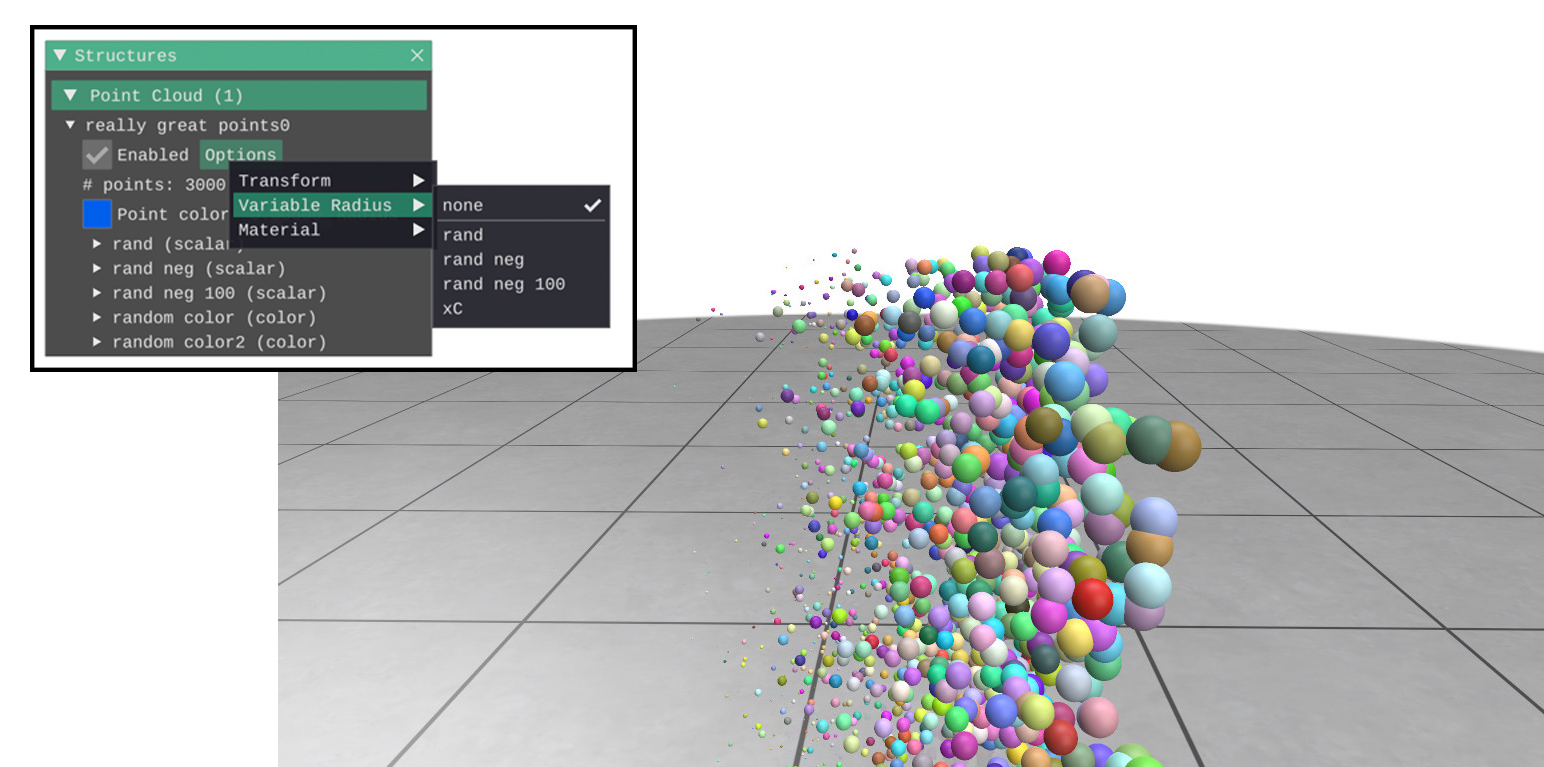By default, all points in the cloud have the same radius. However, any point cloud scalar quantity can be additionally interpreted as the radius of the points. This can also be set manually in the GUI via the point cloud [Options] –> [Variable Radius].Example:

import polyscope as ps
import numpy as np

# ... setup, register a point cloud, etc ...

// Get a reference to your point cloud
ps_cloud = ps.get_point_cloud("your point cloud name")

# Add a random scalar quantity
N = ps_cloud.n_points()
vals = np.random.rand(N)

# Set the quantity as the point size
ps.show()

# Clear it out, go back to default constant size
ps.show()

# Set the quantity as the size using actual world-coordinate units,
# rather than auto-scaling the size
ps.show()


Any negative values in the scalar quantity will be clamped to 0. By default, values will be rescaled such that the largest corresponds to the size from the point radius option (thus, using any constant scalar quantity will make the radii identical to the default value with no radius set). This automatic scaling can be disabled by setting autoscale=False below.

Reproducing radius in world units

Remember that point clouds always have a per-structure radius parameter which sets a radius for all of the points in the point cloud (and can be adjusted via a slider in the GUI, or via radius=0.1/set_radius()). This per-structure parameter makes things a little more complicated when also setting length via a scalar quantity as described here.

By default, the structure radius parameter is still respected. The variable radius from the quantity first scaled such that the largest value is 1., and then is multiplied by the structure parameter to get the actual radius used for the points.

This usually gives a reasonable visualization, but makes it difficult to set a precise radius in world units. To properly reproduce a radius in world-coordinate units, you can circumvent autoscaling like cloud.set_point_radius_quantity(q, autoscale=False). This will prevent the auto-scaling of the radii, and also ignore the structure’s point radius parameter.

PointCloud.set_point_radius_quantity(quantity_name, autoscale=True)
When using a radius which is a physical length in world coordinates, set autoscale to False to skip rescaling and ignore the structure’s point radius parameter.
PointCloud.clear_point_radius_quantity()